# Light: Reflection & Refraction - 2

## 20 Questions MCQ Test Online MCQ Tests for Class 10 | Light: Reflection & Refraction - 2

Description
Attempt Light: Reflection & Refraction - 2 | 20 questions in 20 minutes | Mock test for Class 10 preparation | Free important questions MCQ to study Online MCQ Tests for Class 10 for Class 10 Exam | Download free PDF with solutions
QUESTION: 1

### For a spherical mirror focal length ‘f ’ and radius of curvature ‘R' are correlated as :

Solution:

For a spherical mirror focal length is one half of its radius of curvature i.e. f = R/2 or R = 2f

QUESTION: 2

### In which of the following, the image of an object placed at infinity will be highly diminished and point sized ?

Solution:

The incident ray coming from the object placed at infinity will be parallel to the principal axis. When the parallel beam of light incident on a mirror or lens, irrespective of their nature, after reflection/refraction, will pass or appear to pass through their principal focus. Hence highly diminished and point size image will be formed at their focus.

QUESTION: 3

### When a ray of light travelling parallel to the principal axis of a concave mirror strikes at the polished surface of mirror, the reflected ray

Solution:

When a ray of light travelling parallel to the principal axis of a concave mirror is incident on a concave mirror, the reflected beam passes through its principal focus.

QUESTION: 4

The path of a ray of light coming from air passing through a rectangular glass slab traced by four students are shown as A, B, C and D in figure. Which one of them is correct?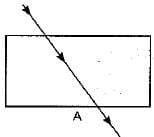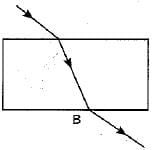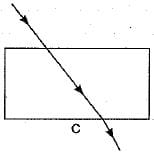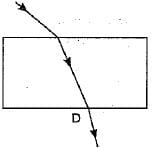Solution:

When a light ray is incident oblikely on one face of rectangular glass slab, the emergent ray will be parallel to the incident ray and shifted sideward slightly.

QUESTION: 5

No matter how far or close you stand from a mirror, your image is always virtual and erect. The mirror is

Solution:

Irrespective of the position of an object both convex mirror and plane mirror form virtual and erect images. Of course image formed by a plane mirror is of same size as the object but image formed by convex mirror is always diminished one.

QUESTION: 6

The laws of reflection hold good for

Solution:

The laws of reflection hold good for light reflected from any smooth surface irrespective of their shapes.

QUESTION: 7

Under which of the following conditions a concave mirror can form a real and inverted image larger than the actual object ?

Solution:

A concave mirror forms a real and inverted image larger than the object size when object is situated between principal focus (F) and centre of curvature (C) of the mirror

QUESTION: 8

In torches, search lights and headlights of vehicles the bulb is placed

Solution:

When source of light is placed verg near to the focus of the reflector which are concave in shape, the reflected light becomes parallel to the principal axis.

QUESTION: 9

Alinear object is placed at a distance equal to focal length of a convex mirror. Its image is formed

Solution:

When a linear object is placed at a distance equal to focal length of a convex mirror then its virtual image is form ed behind the mirror at a distance f/2
As per sign convention u = - f and focal length o f convex mirror is + ve, hence from mirror formula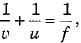we have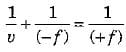or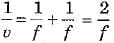or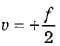QUESTION: 10

A full length image of a distant tall building can definitely be seen by using

Solution:

Convex mirror has a wide field of view as it forms virtual, erect and diminished image. Hence, fall length of image of distance tall building can definitely be seen by using it.

QUESTION: 11

As per the cartesian sign convention followed for mirrors and lenses

Solution:

As per the new Cartesian sign convention followed focal length of a concave mirror as well as of a concave lens is taken with negative sign.

QUESTION: 12

Magnification produced by a rear view mirror fitted in vehicles

Solution:

Convex mirror is used as rear view mirror and alway forms virtual, erect and diminished inage. So magnification produced by a rear view mirror is less than one.

QUESTION: 13

In a convex mirror for any position of the object in front of mirror, the image formed is

Solution:

For a convex mirror irrespective of the position of object the image formed is virtual, erect and diminished one. Moreover, the image is always situated between pole and principal focus of the mirror.

QUESTION: 14

Which of the following statements is/are true?

Solution:

Positive sign with power and focal length indicates that the given lens is convex.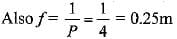QUESTION: 15

An object is placed at a distance of 15 cm from a concave mirror and its real image is formed at 30 cm in front of that mirror. The focal length of the mirror is

Solution:

Here u = - 15 cm and v = - 30 cm, hence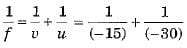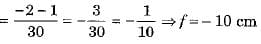QUESTION: 16

A beam of light is incident through the holes on side A and emerges out of the hole on the other face of the box as shown in the figure. Which of the following could be inside the box?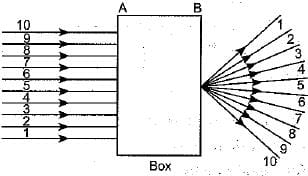Solution:

From figure, it is clear that parallel rays converge at a point and emerges from face B. So convex lens would be possible inside the box.

QUESTION: 17

A ray of light AM is incident on a concave mirror as shown below. Then which of the following ray diagrams is correct for the reflected ray ?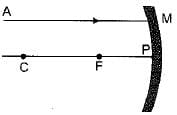Solution:

As the incident light ray is coming parallel to the principal axis of given concave mirror, the reflected ray must pass through the principal focus of mirror.

QUESTION: 18

Beams of light are incident through the holes A and B and emerge out of box through the holes C and D respectively as shown in the figure. Which of the following could be inside the box ?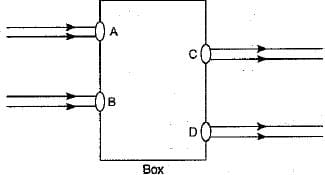Solution:

Figure shows that emergent ray are parallel to the incedent ray and shifted side ward slightly. This can be done by the rectangular glass slab only.

QUESTION: 19

In which of the following, the image of an object placed at infinity will be highly diminished and point sized ?

Solution:

When an object is placed at infinity, a parallel beam of light is incident on the mirror/lens. For all types of mirrors and lenses the beam will get focussed at the principal focus of mirror/lens and a highly diminished, point size image is formed there.

QUESTION: 20

A light ray enters from medium A to medium B as shown in figure. The refractive index of medium B relative to A will be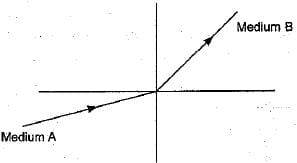Solution:

In medium B, light ray bends away from the normal. It indicates that medium B is optically rarer than medium A. Hence speed of light in medium B is more than that in medium A. Now, refractive index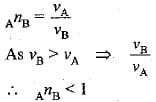Use Code STAYHOME200 and get INR 200 additional OFF Use Coupon Code